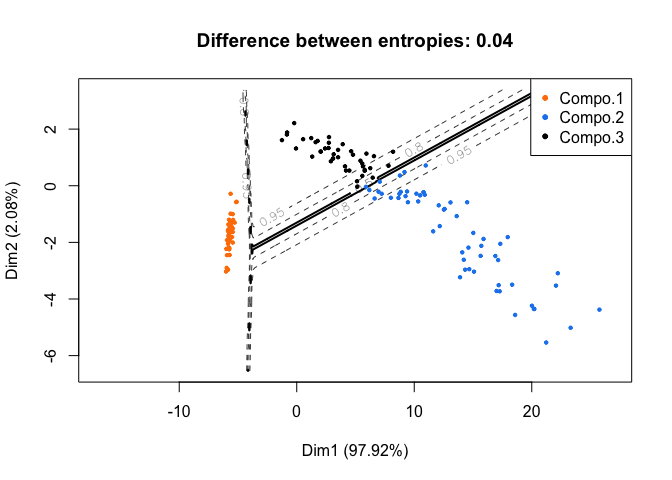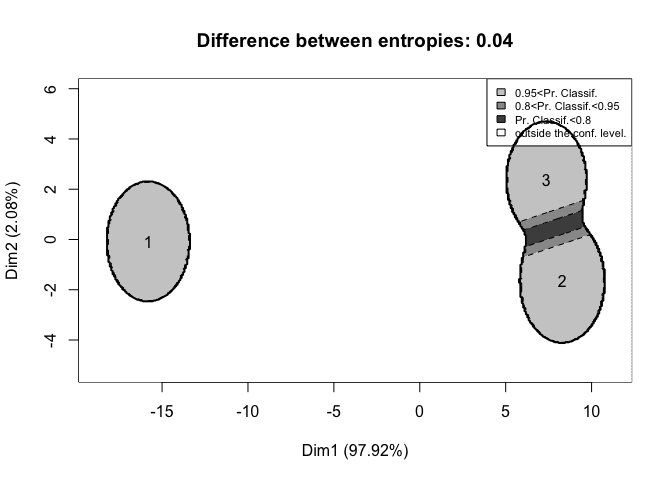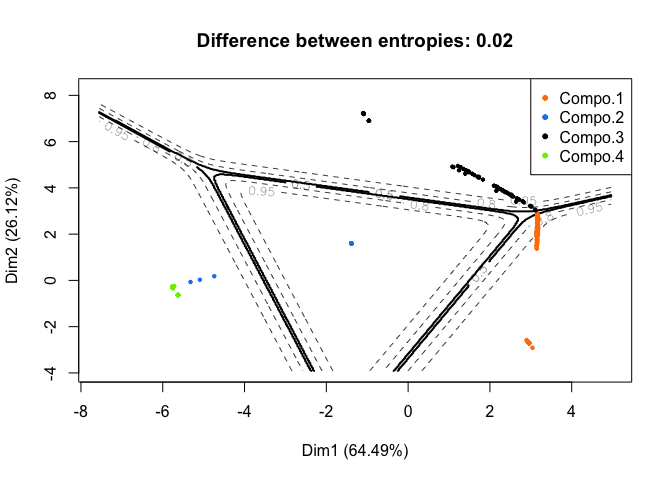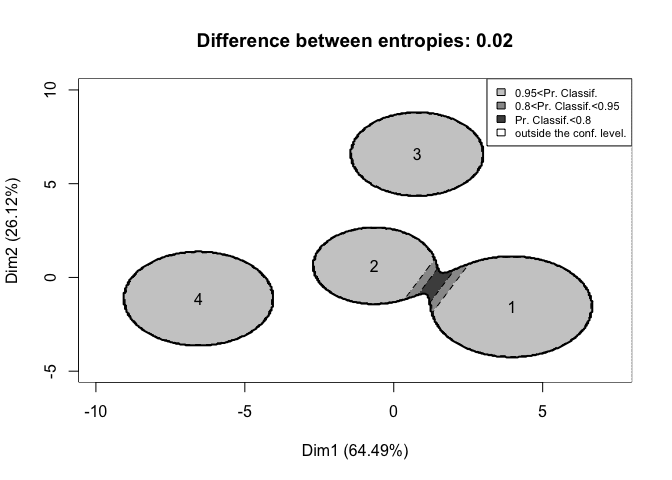Using ClusVis with RMixtComp Output for Visualization

ClusVis

ClusVis is an R package that performs a gaussian-based visualization of gaussian and non-gaussian Model-Based Clustering. This visualization is based on the probabilities of classification. See this preprint for more details about the method. It allows to visualize clusters as bivariate spherical gaussian.

ClusVis and RMixtComp

First, we load the required packages.

library(RMixtComp)
library(ClusVis)

To illustrate the use of ClusVis with RMixtComp output, we use the iris dataset and the congress dataset.

Example 1: iris dataset

The iris dataset gives the measurements in centimeters of the variables sepal length and width and petal length and width, respectively, for 50 flowers from each of 3 species of iris.

data("iris")
##   Sepal.Length Sepal.Width Petal.Length Petal.Width Species
## 1          5.1         3.5          1.4         0.2  setosa
## 2          4.9         3.0          1.4         0.2  setosa
## 3          4.7         3.2          1.3         0.2  setosa
## 4          4.6         3.1          1.5         0.2  setosa
## 5          5.0         3.6          1.4         0.2  setosa
## 6          5.4         3.9          1.7         0.4  setosa

First, we learn a mixture model with 3 classes for the 4 measurements varaibles.

res <- mixtCompLearn(iris[, -5], nClass = 3, criterion = "BIC", nRun = 3, nCore = 1, verbose = FALSE)

Then, we apply the clusvis function. This function requires 2 parameters: the logarithm of the probabilities of classification of every individuals and the proportion of the mixture.

logTik <- getTik(res, log = TRUE)
prop <- getProportion(res)
resVisu <- clusvis(logTik, prop)

The results can be displayed using the plotDensityClusVisu function. The first graph is generated with the parameter add.obs = TRUE. It overlays on the most discriminative map the curve of iso-probabilities of classification and the cloud of observations.With add.obs = FALSE, the goal of the plot is to represents the overlap between the clusters. Each clusters is represented by its centers and a 95% confidence level border. The differene between entropies displayed in the title defines the accuracy of the representation. A difference closed to 0 means that the representation is accurate.Here, we note that two clusters are closed and so they contains flowers with similar measures whereas the other cluster contains flowers with very different measures from the two others.

Example 2: congress dataset

This data set includes votes for each of the U.S. House of Representatives Congressmen on the 16 key votes identified by the CQA in 1984.

data("congress")
##           V1 V2 V3 V4 V5 V6 V7 V8 V9 V10 V11 V12 V13 V14 V15 V16 V17
## 1 republican  n  y  n  y  y  y  n  n   n   y   ?   y   y   y   n   y
## 2 republican  n  y  n  y  y  y  n  n   n   n   n   y   y   y   n   ?
## 3   democrat  ?  y  y  ?  y  y  n  n   n   n   y   n   y   y   n   n
## 4   democrat  n  y  y  n  ?  y  n  n   n   n   y   n   y   n   n   y
## 5   democrat  y  y  y  n  y  y  n  n   n   n   y   ?   y   y   y   y
## 6   democrat  n  y  y  n  y  y  n  n   n   n   n   n   y   y   y   y

First, we change the format of the data. The vote “n” is refactored as 1 and “y” as 2. “democrat” is refactored as 1 and “republican” as 2.

## MixtComp Format
congress$V1 = refactorCategorical(congress$V1, c("democrat", "republican", "?"), c(1, 2, "?"))
for(i in 2:ncol(congress))
congress[, i] = refactorCategorical(congress[, i], c("n", "y", "?"), c(1, 2, "?"))

##   V1 V2 V3 V4 V5 V6 V7 V8 V9 V10 V11 V12 V13 V14 V15 V16 V17
## 1  2  1  2  1  2  2  2  1  1   1   2   ?   2   2   2   1   2
## 2  2  1  2  1  2  2  2  1  1   1   1   1   2   2   2   1   ?
## 3  1  ?  2  2  ?  2  2  1  1   1   1   2   1   2   2   1   1
## 4  1  1  2  2  1  ?  2  1  1   1   1   2   1   2   1   1   2
## 5  1  2  2  2  1  2  2  1  1   1   1   2   ?   2   2   2   2
## 6  1  1  2  2  1  2  2  1  1   1   1   1   1   2   2   2   2

We run MixtComp with a Multinomial model for each variable.

model <- rep("Multinomial", ncol(congress))
names(model) = colnames(congress)

res <- mixtCompLearn(congress, model = model, nClass = 4, criterion = "BIC", nRun = 3, nCore = 1)

As before, we extract the required parameters.

logTik <- getTik(res, log = TRUE)
prop <- getProportion(res)
##           [,1]       [,2]          [,3]          [,4]
## [1,]      -Inf  -6.858874          -Inf -0.0010506472
## [2,]      -Inf  -8.227312          -Inf -0.0002672894
## [3,] -22.30520       -Inf -2.055760e-10          -Inf
## [4,] -10.90311 -15.973071 -1.851677e-05          -Inf
## [5,] -15.90965 -13.566076 -1.406477e-06          -Inf
## [6,] -14.71938 -10.496007 -2.805201e-05          -Inf

It is important to notice that there are a lot of -Inf values in the variable logTik because some probabilities to be in a cluster are exactly 0. If there are too many infinite values, it is a problem for the cluvis function. One way to avoid this problem is to replace infinite values with the logarithm of a epsilon.

logTik[is.infinite(logTik)] = log(1e-20)
##           [,1]       [,2]          [,3]          [,4]
## [1,] -46.05170  -6.858874 -4.605170e+01 -1.050647e-03
## [2,] -46.05170  -8.227312 -4.605170e+01 -2.672894e-04
## [3,] -22.30520 -46.051702 -2.055760e-10 -4.605170e+01
## [4,] -10.90311 -15.973071 -1.851677e-05 -4.605170e+01
## [5,] -15.90965 -13.566076 -1.406477e-06 -4.605170e+01
## [6,] -14.71938 -10.496007 -2.805201e-05 -4.605170e+01

Now, the clusvis function can be run.

resVisu <- clusvis(logTik, prop)

And the two associated plots generated.